# php tutorial - PHP While Loop - php programming - learn php - php code - php script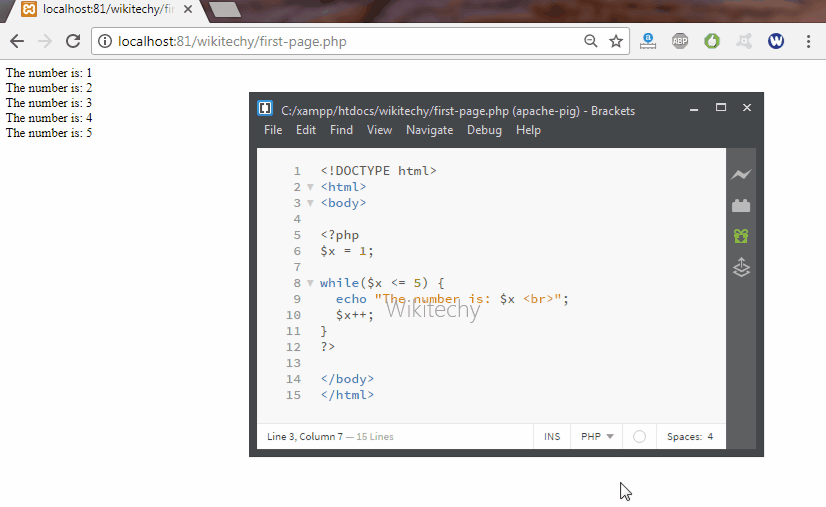Learn PHP - PHP tutorial - While Loop - PHP examples - PHP programs

• While loops will be executed if the set of code for the particular condition is true.
• If the condition becomes false, the statements within the loop stops executing and control passes to the next statement in the loop.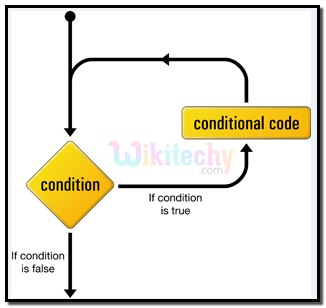## php scripts Syntax :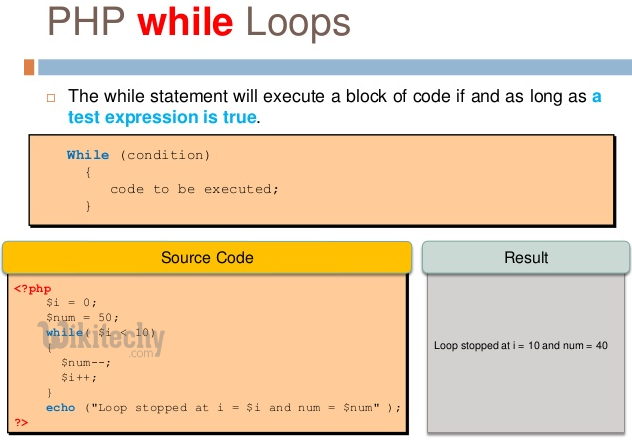## php programmer Code Explanation :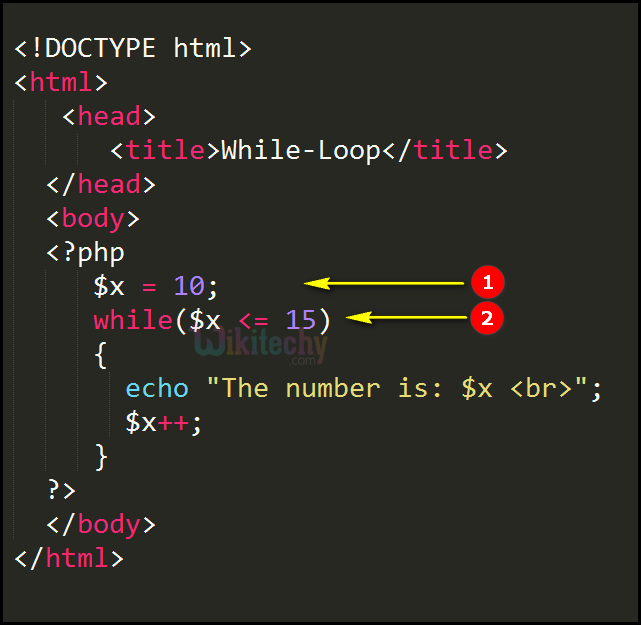1. \$x =10 is a variable.
2. while(\$x <= 15) is the while loop which will continue to run as long as \$x=10 is less than, or equal to 15 (\$x <= 15). \$x =10 will increase by 1 each time the loop runs (\$x++) variable till 15.

## php development Sample Output :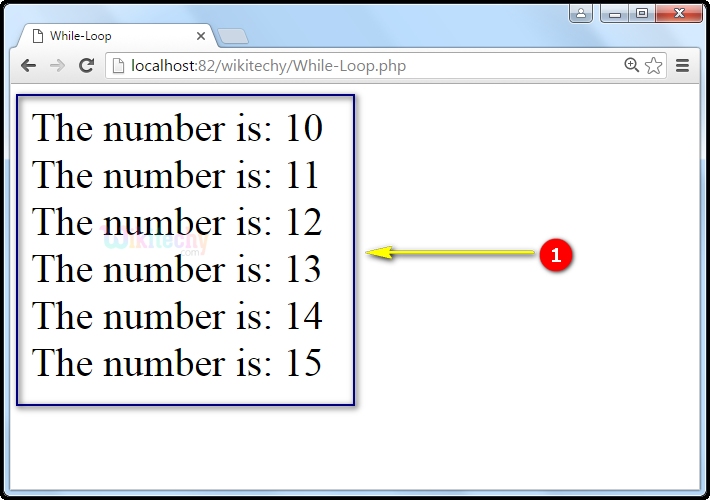1. While loop will continue to run as long as \$x=10 is less than, or equal to 15 (\$x <= 15) which will increase by 1 each time the loop runs (\$x++) till 15.